CYa_暂退

CYa_暂退
6天前CYa_暂退
7天前

C++。dfs，回溯+剪枝。。。就我觉得中等题做到后面，完成一道题花的时间越来越长吗？下面说下思路

1. 用left和right变量控制ip地址每段的范围，其中：
• left作为每段起始点，且在每次函数调用中向后递进，即dfs(ans, s, depth + 1, right + 1, temp + ts + “.”);；
• righr作为每段终点，同时也是循环变量，调整每段的长度和大小；
• right作为当前段终点，如果剩下的ip段长度不符合要求（即剩下的每段长度必须在1-3之间），则舍弃；
2. temp变量记录到当前位置，已经转化好的ip字符串；
3. depth表示当前是ip中第几段；

- 每段ip的要求不仅是0-255，同时每段不得有前置零，且不得删除任何一个数，即ip地址总长度不能变
- 字符串与数值的转化，虽然写起来不难，但已经有现成的函数可用。。。sscanf和sprintf了解一下
- 函数形参声明为引用，能提高效率

class Solution {
public:
vector<int> ans;
vector<int> inorderTraversal(TreeNode* root) {
dfs(root);
return ans;
}

void dfs(TreeNode* root) {
if (!root) return;
dfs(root->left);
ans.push_back(root->val);
dfs(root->right);
}
};


CYa_暂退
7天前

$O(N)$

class Solution {
public:
int numDecodings(string s) {
int n = s.size();
s = ' ' + s;
vector<int> f(n+1);
f = 1;
for (int i = 1; i <= n; i++){
if (s[i] >= '1' && s[i] <= '9' ) f[i] += f[i-1];
if (i > 1){
int t = (s[i - 1] - '0') * 10 + s[i] - '0';
if (t >= 10 && t <= 26) f[i] += f[i - 2];
}
}
return f[n];
}
};


python

class Solution:
def numDecodings(self, s: str) -> int:
size = len(s)
if size == 0:
return 0

# dp[i]：以 s[i] 结尾的前缀字符串的解码个数

# 分类讨论：
# 1、s[i] != '0' 时，dp[i] = dp[i - 1]
# 2、10 <= s[i - 1..i] <= 26 时，dp[i] += dp[i - 2]
dp = [0 for _ in range(size)]

if s == '0':
return 0
dp = 1

for i in range(1, size):
if s[i] != '0':
dp[i] = dp[i - 1]

num = 10 * (ord(s[i - 1]) - ord('0')) + (ord(s[i]) - ord('0'))

if 10 <= num <= 26:
if i == 1:
dp[i] += 1
else:
dp[i] += dp[i - 2]
return dp[size - 1]



CYa_暂退
7天前

### 方法一: 递归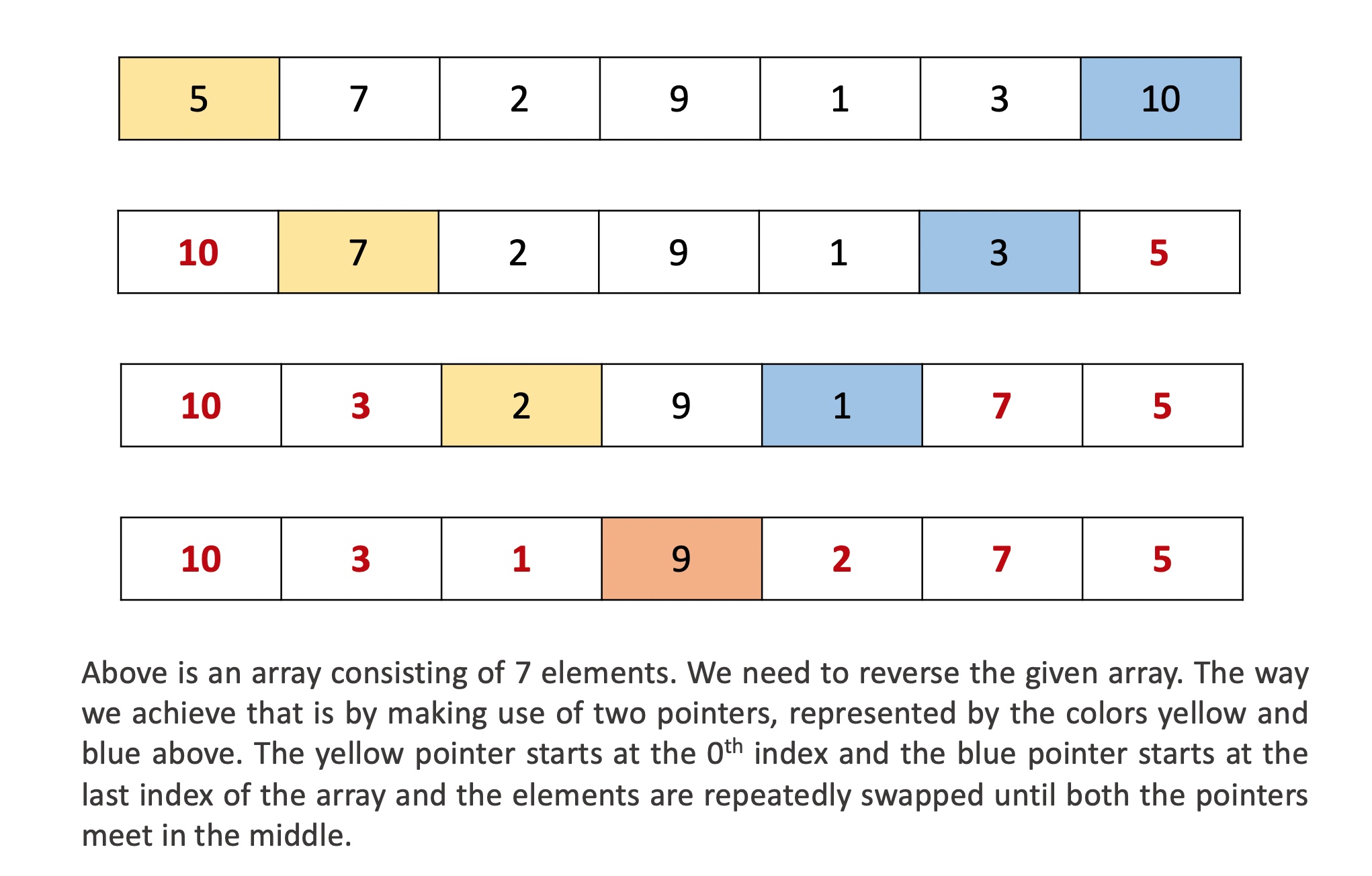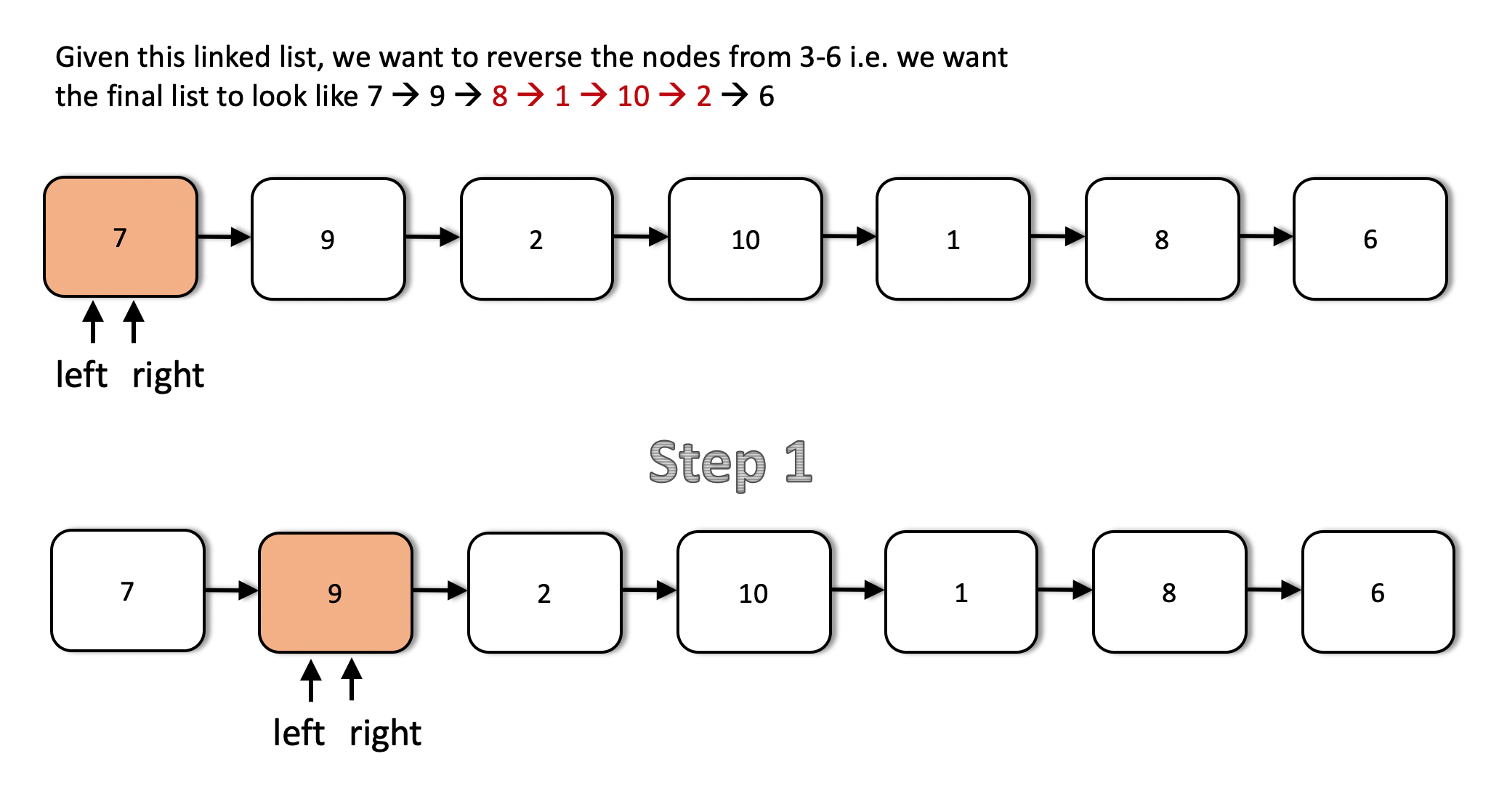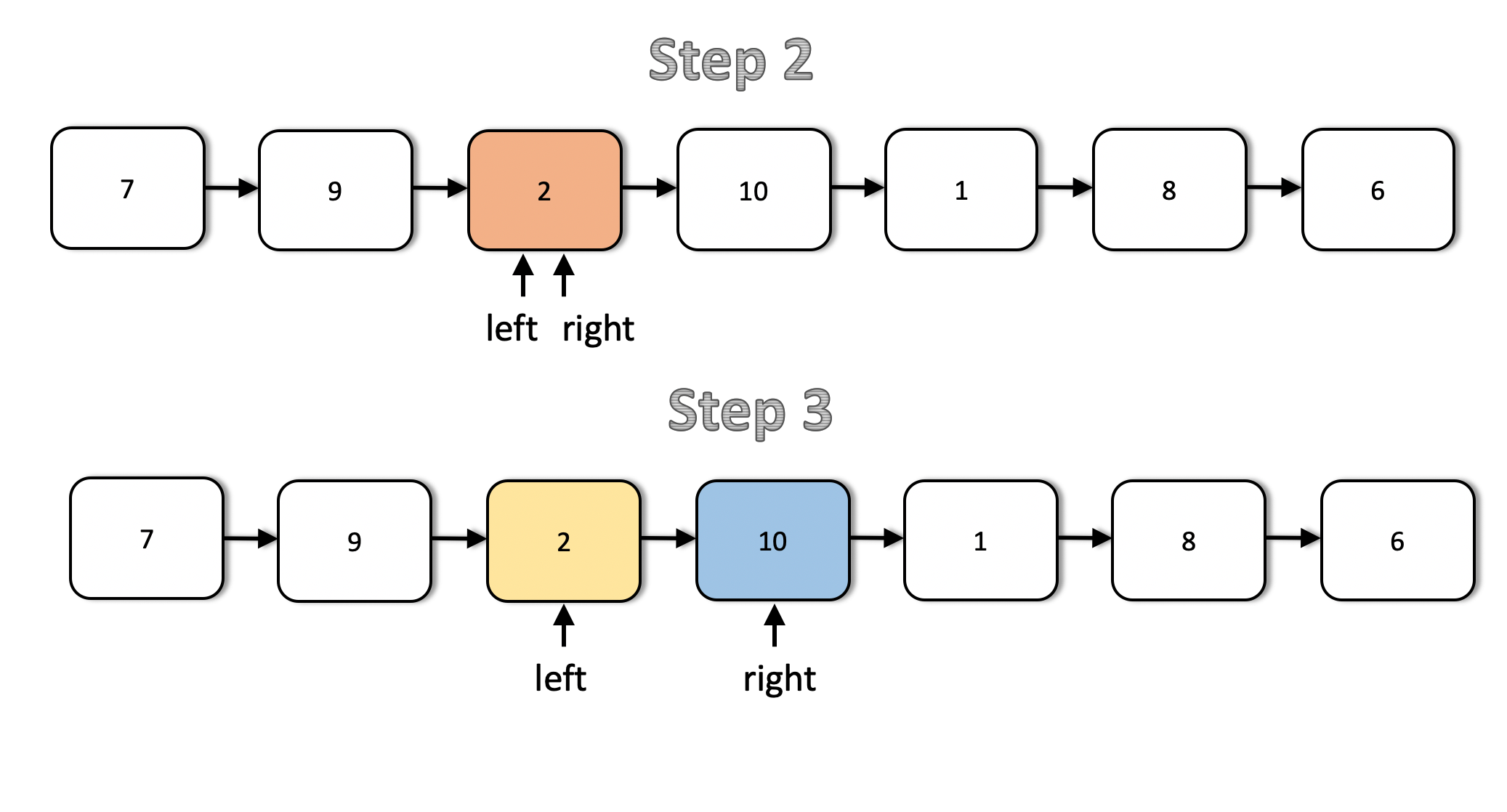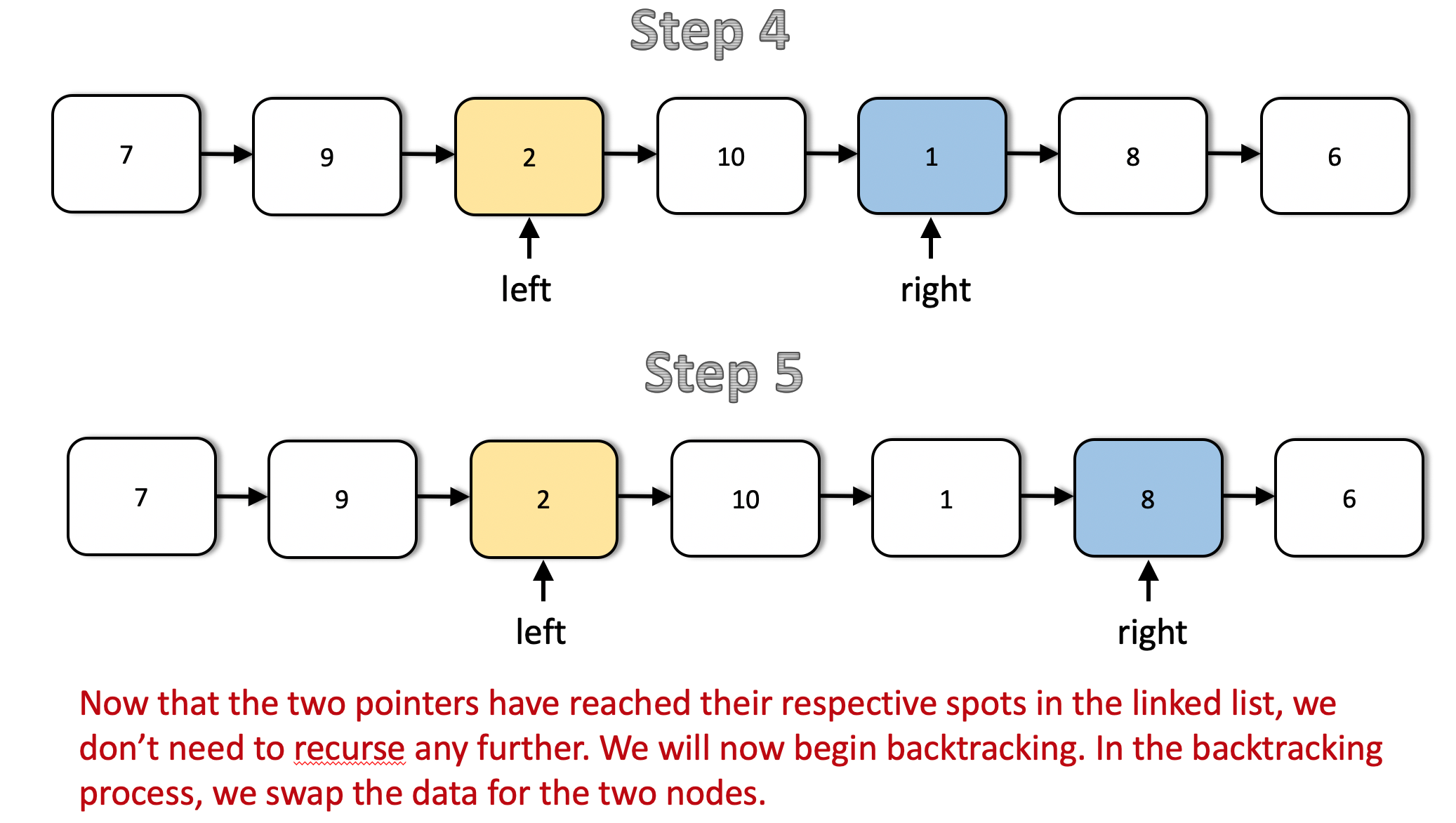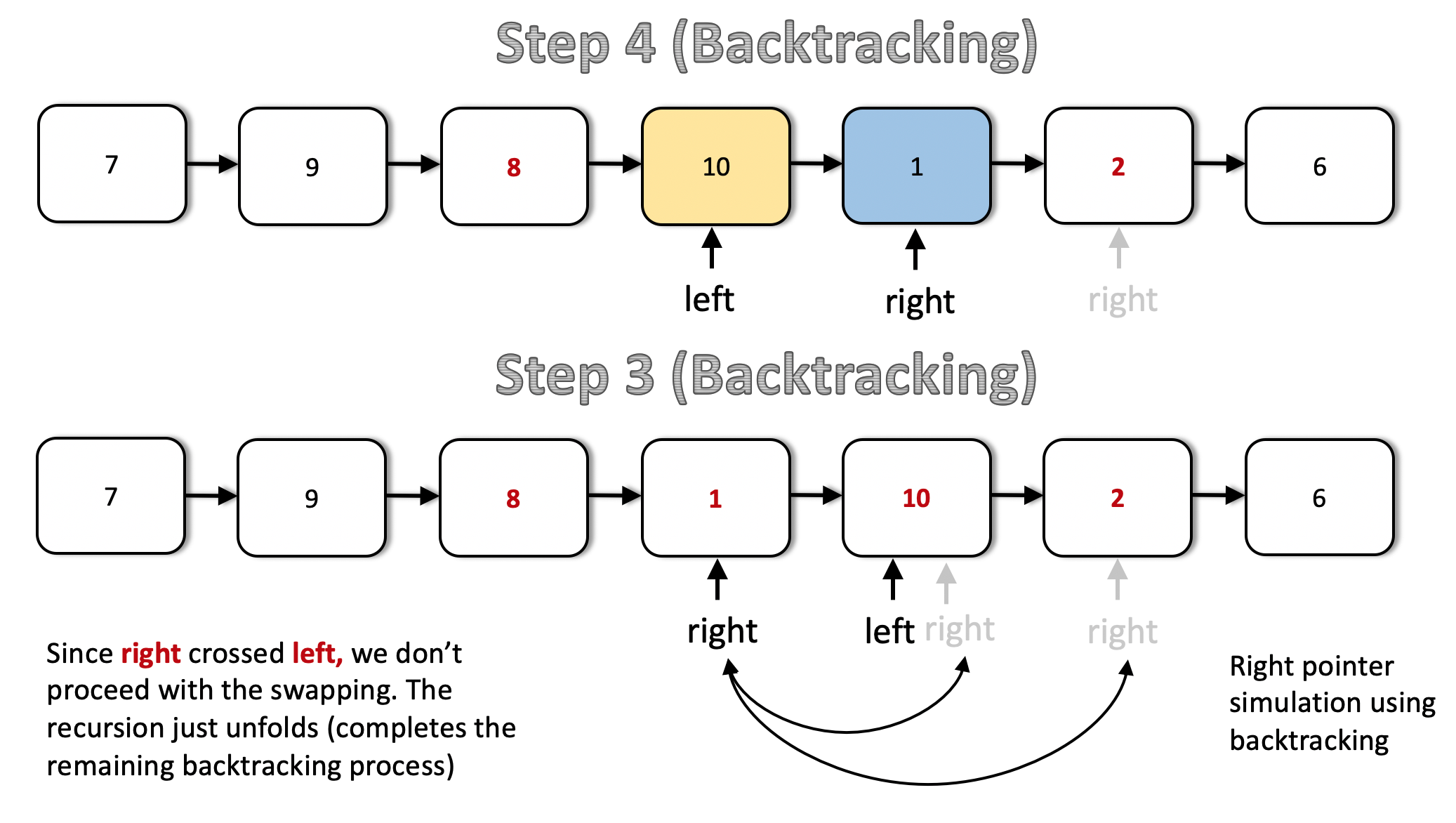class Solution:
"""
:type m: int
:type n: int
:rtype: ListNode
"""
return None

stop = False
def recurseAndReverse(right, m, n):
nonlocal left, stop

# base case. Don't proceed any further
if n == 1:
return

# Keep moving the right pointer one step forward until (n == 1)
right = right.next

# Keep moving left pointer to the right until we reach the proper node
# from where the reversal is to start.
if m > 1:
left = left.next

# Recurse with m and n reduced.
recurseAndReverse(right, m - 1, n - 1)

if left == right or right.next == left:
stop = True



$c++$

class Solution {
public:
ListNode* reverseBetween(ListNode* head, int m, int n) {
auto dummy = new ListNode(-1);

auto a = dummy;
for (int i = 0; i < m - 1; i ++ ) a = a->next;
auto b = a->next, c = b->next;
for (int i = 0; i < n - m; i ++ ) {
auto t = c->next;
c->next = b;
b = c, c = t;
}
a->next->next = c;
a->next = b;
return dummy->next;
}
};


CYa_暂退
7天前

Definition: 书上: 编写表示现实世界中的事物和情景，并基于这些类来创建对象。定义一大类都有的通用行为。e.g.:基于类创建对象时，每个对象都自动具备这种通用行为。根据类来创建对象被称为实例化

1. 视频定义: 把一组数据结构和处理他们的方法组成对象。把具有$\underline{相同行为}$的对象归纳为。通过封装隐藏类的内部1细节。通过继承是类得到泛化。通过多态实现基于对象类型的动态分派。
2. 分别解析7个关键词：

1) 数据 加工后变成信息
2) 方法 为了达成某种目的而采取的途径步骤和手段。如果把函数定义为类的一部分或将函数与某个对象绑定，该函数称之为方法
3) 对象 特定的人或物 在计算机中的逻辑映射
4) 类class Ch9_P138 引用数据类型： 数据说明+ 操作数据的方法 e.g.:人类 实例:我们每个人，即对象。包含性别年龄等数据说明 + 方法: 走路工作吃饭 传递能量的方法
5) 封装 对某一类事物的抽象描述 通过将姓名性别年龄等属性抽象到人类这个类类型的过程即为封装。一说到人就会联系到人的名字，是男是女，+隐藏属性：身上多少钱
6) 继承 P147 子类__init__ 父类 使得子类获得子类获得父类公开的属性和方法 同时可以拓展自身所拥有额属性和方法
7) 多态 接口：特殊的类，指定必须做什么。(无需告诉怎么做) 用多态实现接口- 如:吃饭 类A:去餐厅筷子吃鱼 类B: 去西餐厅刀叉吃牛排

class ClassName:
<statement-1>
.
.
.
<statement-N>


#!/usr/bin/python3

class MyClass:
"""一个简单的类实例"""
i = 12345
def f(self):
return 'hello world'

# 实例化类
x = MyClass()

# 访问类的属性和方法
print("MyClass 类的属性 i 为：", x.i)
print("MyClass 类的方法 f 输出为：", x.f())



MyClass 类的属性 i 为： 12345
MyClass 类的方法 f 输出为： hello world

def __init__(self):
self.data = []


x = MyClass()


#!/usr/bin/python3

class Complex:
def __init__(self, realpart, imagpart):
self.r = realpart
self.i = imagpart
x = Complex(3.0, -4.5)
print(x.r, x.i)   # 输出结果：3.0 -4.5


CYa_暂退
8天前CYa_暂退
10天前CYa_暂退
10天前

#include <iostream>
using namespace std;

const int N = 100010;
int A[N], B[N], C[N];

int Add(int a[], int b[], int c[], int cnt) {

int t = 0;//t表示进位

for (int i=1; i<=cnt; i++) {
t += a[i] + b[i];//进位加上a和b第i位上的数
c[i] = t % 10;//c的值就是进位的个位数
t /= 10;//把t的个位数去掉只剩下十位数，即只剩下这个位置的进位
}
if (t) c[++cnt] = 1;//如果t==1，表示还有一个进位，要补上

return cnt;
}

int main() {

string a, b;
cin >> a >> b;

//A和B倒着放进int数组，因为有进位，倒着放容易处理
int cnt1 = 0;
for (int i=a.size()-1; i>=0; i--)
A[++cnt1] = a[i] - '0';

int cnt2 = 0;
for (int i=b.size()-1; i>=0; i--)
B[++cnt2] = b[i] - '0';

int tot = Add(A, B, C, max(cnt1, cnt2));

//因为A和B是倒着放的，所以C也要倒着输出
for (int i=tot; i>=1; i--)
cout << C[i];
}


CYa_暂退
10天前

### 算法

#### (暴力枚举) $O(n2^n)$ 8ms

class Solution {
public:
vector<vector<int>> ans;
vector<int> path;

vector<vector<int>> subsetsWithDup(vector<int>& nums) {
sort(nums.begin(), nums.end());
dfs(0, nums);
return ans;
}
void dfs(int u, vector<int>&nums)
{
if (u == nums.size())
{
ans.push_back(path);
return;
}
int k = u;
while (k < nums.size() && nums[k] == nums[u]) k ++ ;
dfs(k, nums);
for (int i = u; i < k; i ++ )
{
path.push_back(nums[i]);
dfs(k, nums);
}
path.erase(path.end() - (k - u), path.end()); //erase这里是回溯里的恢复现场操作，递归结束后需要将path数组恢复原状
}
};


y总

class Solution {
public:

vector<vector<int>> ans;
vector<int> path;

vector<vector<int>> subsetsWithDup(vector<int>& nums) {
sort(nums.begin(), nums.end());
dfs(nums, 0);
return ans;
}

void dfs(vector<int>& nums, int u) {
if (u == nums.size()) {
ans.push_back(path);
return;
}

int k = u + 1;
while (k < nums.size() && nums[k] == nums[u]) k ++ ;

for (int i = 0; i <= k - u; i ++ ) {
dfs(nums, k);
path.push_back(nums[u]);
}

for (int i = 0; i <= k - u; i ++ ) {
path.pop_back();
}
}
};


python版

# @param num, a list of integer
# @return a list of lists of integer
class Solution:
def subsetsWithDup(self, S):
res = [[]]
S.sort()
for i in range(len(S)):
if i == 0 or S[i] != S[i - 1]:
l = len(res)
for j in range(len(res) - l, len(res)):
res.append(res[j] + [S[i]])
return res


CYa_暂退
10天前

### 算法1 (递归)

#### 题解1: T-SHLoRk

class Solution {
public:
vector<int> grayCode(int n) {
vector<int> res;
res.push_back(0);
int t = 1,size = 1;
for(int k = 0 ; k < n ; k ++)
{
vector<int> cur;
for(int i = 0 ; i < size ;i ++)
cur.push_back(res[i]);
for(int i = size - 1; i >= 0 ; i --)
cur.push_back(res[i] + t);
res = cur;
size = res.size();
t = t << 1;
}
return res;
}
};


1.5 y总 双摆算法：

class Solution {
public:
vector<int> grayCode(int n) {
vector<int> res(1, 0);
while (n -- ) {
for (int i = res.size() - 1; i >= 0; i -- ) {
res[i] *= 2;
res.push_back(res[i] + 1);
}
}
return res;
}
};


### 2 (异或生成) 0ms 100% ！！

vector<int> grayCode(int n) {
int count = 1 << n;
vector<int> res(count,0);
for(int i = 1 ; i < count; i ++)
{
int bin = i,cur = bin >> (n - 1);
for(int k = n - 1;k > 0;k --)
cur = (cur << 1) + (((bin >> k) & 1) ^ ((bin >>(k - 1)) & 1));
res[i] = cur;
}
return res;
}


python版·56ms

def grayCode(self, n):
results = 
for i in range(n):
results += [x | pow(2, i) for x in reversed(results)]
return results


start: 
i = 0:          
i = 1:          [0, 1]
nums = nums + 1
i = 2:          [0, 1, 3, 2]
nums[2:4] = nums[1: : -1] + 2
i = 3:          [0, 1, 3, 2, 6, 7, 5 ,4]
nums[4:8] = nums[3:: -1] + 4